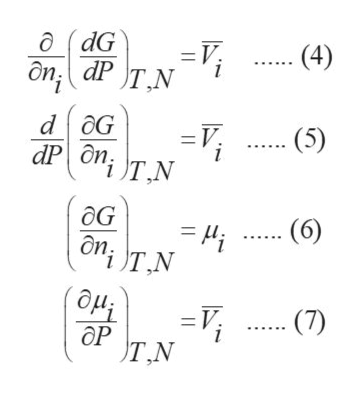# 8.4. Rigorously show that (aV/oN)T,P of Eqn. (8.7) is equal to V/N. (8.7)aNT,PОР/ т,N

Question
66 views

This problem is (8.4) from a book  "Thermodynamics and Statistical Mechanics An Integrated Approach by M. Scott Shell"

check_circle

Step 1

The change in Gibb’s free energy for a reversible process is given by equation (1). At, constant temperature, equation (1) reduces to equation (2) as dT is equated to zero. Equation (2) can rearranged and written as equation (3).

Step 2

On differentiating equation (3) with respect to number of moles of component i, equation (4) is obtained where Vi bar is partial molar volume or V/N of component i. Equation (4) is rewritten as equation (5) on rearrangement. It is known that the partial change in Gibb’s free energy with respect to the moles of component i at constant T a...help_outlineImage Transcriptioncloseд (dG дn, dP т.N (4) dOG V. -и аP дn, т.N (5) ! (6) ЕM дn; т.N (ди (7) ОР Т.N fullscreen

### Want to see the full answer?

See Solution

#### Want to see this answer and more?

Solutions are written by subject experts who are available 24/7. Questions are typically answered within 1 hour.*

See Solution
*Response times may vary by subject and question.
Tagged in

### Chemical Engineering